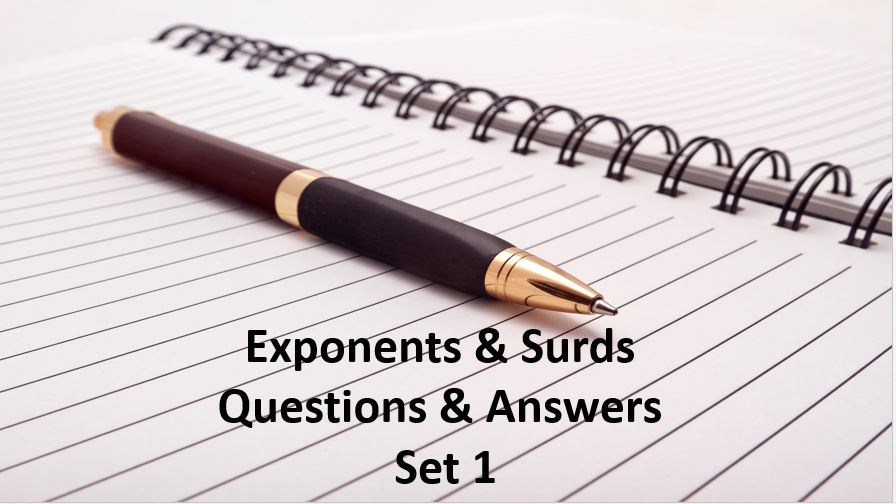Monday, September 27, 2021
Home > Question and Answer > Exponents And Surds Questions And Answer Set 1

# Exponents And Surds Questions And Answer Set 1# Exponents And Surds Questions and Answer Set 1

Hi students, welcome to Amans Maths Blogs (AMB). On this post, you will get the Exponents And Surds Questions and Answer Set 1 is the collection of some important questions. Practice these questions for SSC CGL CHSL CAT NTSE exams etc. It will help you to practice the questions on the topics of maths as exponents and surds based questions of algebra.

Exponents And Surds Questions and Answer Set 1: Ques No 1

The expressioncan be rewritten as

Options:

A. x12/16

B. x15/16

C. x24/16

D. x31/16

Exponents And Surds Questions and Answer Set 1: Ques No 2

If 100.48 = x, 100.70 = y and xz = y2, then the value of z is

Options:

A. 35/10

B. 25/36

C. 35/12

D. 27/16

Exponents And Surds Questions and Answer Set 1: Ques No 3

The simplification ofis

Options:

A. 1

B. 2

C. (b – a)/(a – b)

D. 1/ab

Exponents And Surds Questions and Answer Set 1: Ques No 4

If 6a = 2, 6b = 5 and 6c = 15, then the value of c in terms of a and/or b is

Options:

A. b + 3

B. 5a + b

C. b2 – 2b

D. b – a + 1

Exponents And Surds Questions and Answer Set 1: Ques No 5

If a2+b2+c2 = ab+bc+ca, then the simplification of [xa/xb]a-b * [xb/xc]b-c * [xc/xa]c-a is

Options:

A. 0

B. 1

C. x

D. 1/x

Exponents And Surds Questions and Answer Set 1: Ques No 6

If 3x-y = 81 and 3x+y = 729, then the value of x is

Options:

A. 1

B. 5

C. 2

D. 4

Exponents And Surds Questions and Answer Set 1: Ques No 7

The value ofis

Options:

A. 1.00

B. 1.25

C. 1.50

D. 1.75

Exponents And Surds Questions and Answer Set 1: Ques No 8

The simplification ofis

Options:

A. 1

B. 4

C. 2n

D. 1/2n

Exponents And Surds Questions and Answer Set 1: Ques No 9

If log2 4 * log4 8 * log8 16 * ……………nth term = 49, then the value of n is

Options:

A. 49

B. 48

C. 34

D. 24